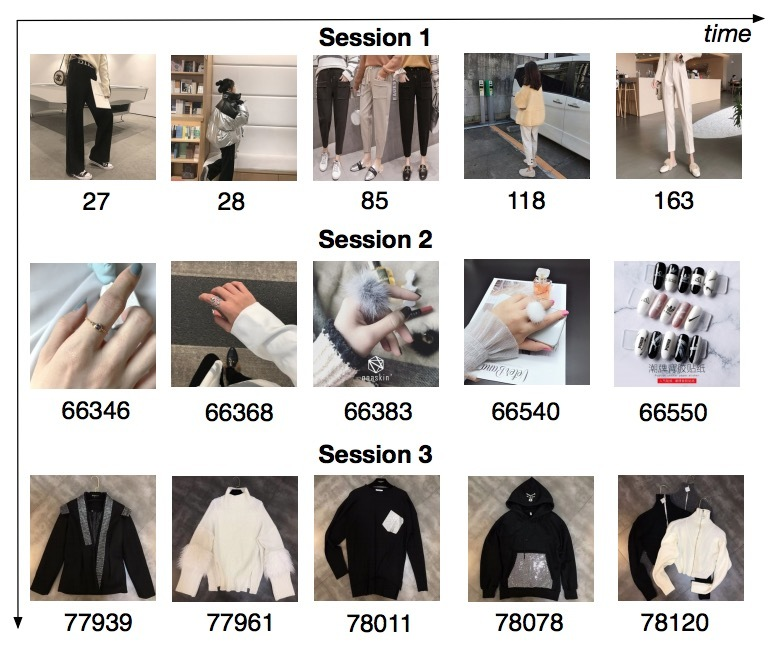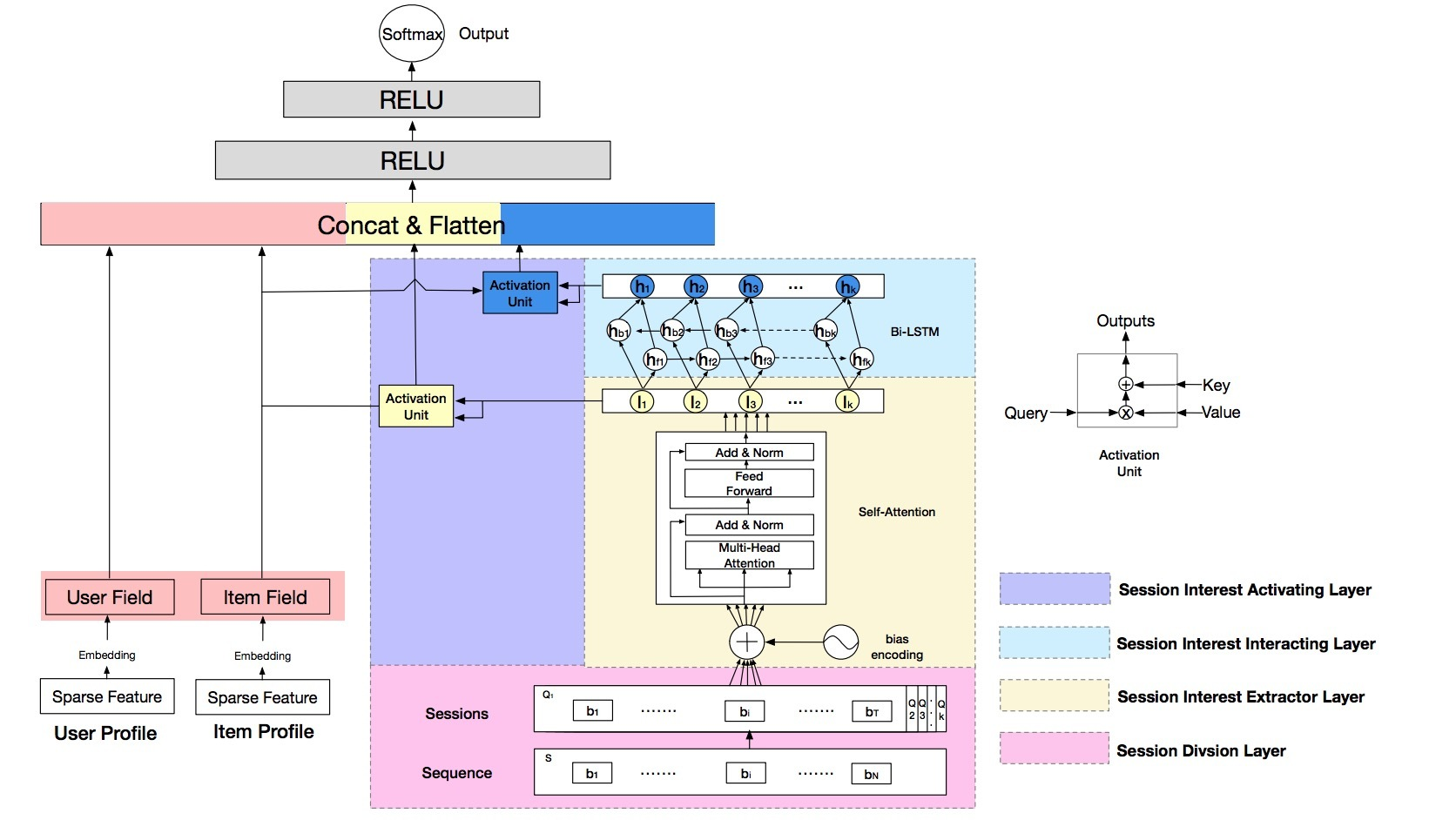1.介绍• 首先，将用户序列行为划分成sessions
• 接着，使用self-attention network以及bias encoding来建模每个session。Self-attention可以捕获session行为(s)的内在交互/相关，接着抽取每个session的用户兴趣(s)。这些不同的session interests可能相互间相关，接着遵循一个序列模式。
• 最后，我们使用Bi-LSTM来捕获交互、以及用户多个历史session interests的演进。由于不同session interests对于target item具有不同的影响，最终我们设计了local activation unit根据target item来聚合他们，形成该行为序列的最终表示。

• 我们强调用户行为在每个session中高度同质，不同sessions差异很大。
• 设计了一个self-attention network以及bias encoding来获得每个session的精准兴趣表示。接着我们使用Bi-LSTM来捕获历史sessions间的顺序关系(sequential relationship)。最后，考虑到不同session interest在target item上的影响，我们使用local activation unit来聚合。
• 两组比较实验。表明DSIN效果更好。

2.相关工作

2.2 Session-based推荐

session的概率常被序列化推荐提及，但很少出现在CTR预测任务中。Session-based推荐受益于用户兴趣在sessions上动态演化的启发。GFF使用关于items的sum pooling来表示一个session。每个item具有两种表示，一个表示自身，另一个表示session的上下文(context)。最近，RNN-based方法被应用于session-based推荐中来捕获在一个session中的顺序关系。基于此，Li 2017提出了一个新的NARM（attentive neural networks framework）来建模用户的序列化行为，并能捕获用户在当前session中的主要目的。Quadrana 2017提出的Hierarchical RNN依赖于RNNs的latent hidden states跨用户历史sessions的演化。另外，Liu 2018 的RNNs使用self-attention based模型来有效捕获一个session的long-term和short-term兴趣。Tang 2018使用CNN、Chen 2018使用user memory network来增强序列模型的表现力。

3.DSIN

3.1 BaseModel

• feature representation,
• embedding,
• MLP以及loss function。

CTR预测任务中统计了大量信息特征。总共使用了三大组：User profile、item profile、User Behavior。每组包含了一些稀疏特征：

• User Profile包含了gender、city等；
• Item Profile包含了：seller id、brand id等；
• User Behavior包含了用户最近点击的item ids等

Embedding

MLP

Loss Function

3.2 模型总览• 1) session division layer，会将用户行为序列划分为sessions
• 2) session interest extractor layer：会抽取用户的session interests
• 3) session interest interacting layer：会捕获session interests间的顺序关系
• 4) session interest activating layer：会对与target item有关的session interests使用local activation unit

1.Session Division Layer

$Q_k = [b_1; \cdots; b_i; \cdots; b_T] \in R^{T \times d_{model}}$

• T是我们在该session中的行为数
• $b_i$是在该session中的用户第i个行为

2.Session Interest Extractor Layer

2-1 Bias Encoding

$BE \in R^{K \times T \times d_{model}}$

$BE_{(k,t,c)} = w_k^K + w_t^T + w_c^C$

…(2)

• $w^K \in R^K$：是session的bias vector
• k：是sessions的索引
• $w^T \in R^T$：是在session中position的bias vector
• t：是在sessions中行为的索引
• $w^C \in R^{d_{model}}$：是在behavior embedding中unit position的bias vector
• c：是在behavior embedding中unit的index

$Q = Q + BE$

…(3)

$Q_k = [Q_{k1}; \cdots; Q_{kh}; \cdots; Q_{kH}]$

• $Q_{kh} \in R^{T \times d_h}$：是$Q_k$的第h个head
• $d_h = \frac{1}{h} d_{model}$

$head_h$的输出如下计算：

$head_h = Attention(Q_{kh} W^Q, Q_{kh} W^K, Q_{kh} W^V) \\ =softmax(\frac{Q_{kh} W^Q W^{K^T} Q_{kh}^T}{\sqrt{d_{model}}}) Q_{kh} W^V$

…(4)

$I_k^Q = FFN(Concat(head_1, \cdots, head_H) W^O)$

…(5)

$I_k = Avg(I_k^Q)$

…(6)

3.Session Interest Interacting Layer

$i_t = \sigma(W_{xi} I_t + W_{hi} h_{t-1} + W_{ci} c_{t-1} + b_i) \\ f_t = \sigma(W_{xf} I_t + W_{hf} h_{t-1} + W_{cf} c_{t-1} + b_f) \\ c_t = f_t c_{t-1} + i_t tanh(W_{xc}I_t + W_{hc} h_{t-1} + b_c) \\ o_t = \sigma(W_{xo} I_t + W_{ho} h_{t-1} + W_{co}c_t + b_o) \\ h_t = o_t tanh(c_h)$

…(7)

$H_t = \overrightarrow {h_{ft}} \oplus \overleftarrow {h_{bt}}$

…(8)

4.Session Interest Activating Layer

$a_k^I = \frac{exp(I_k W^I X^I)}{\sum\limits_k^K exp(I_k W^I X^I)} \\ U^I = \sum\limits_k^K a_k^I I_k$

…(9)

$a_k^H = \frac{exp(H_k W^H X^I)}{\sum\limits_k^K exp(H_k W^H X^I)} \\ U^H = \sum\limits_k^K a_k^H H_k$

…(10)

参考

Updated on

STAR算法介绍

PLE介绍

Published on March 04, 2021

DTS介绍

Published on January 02, 2021